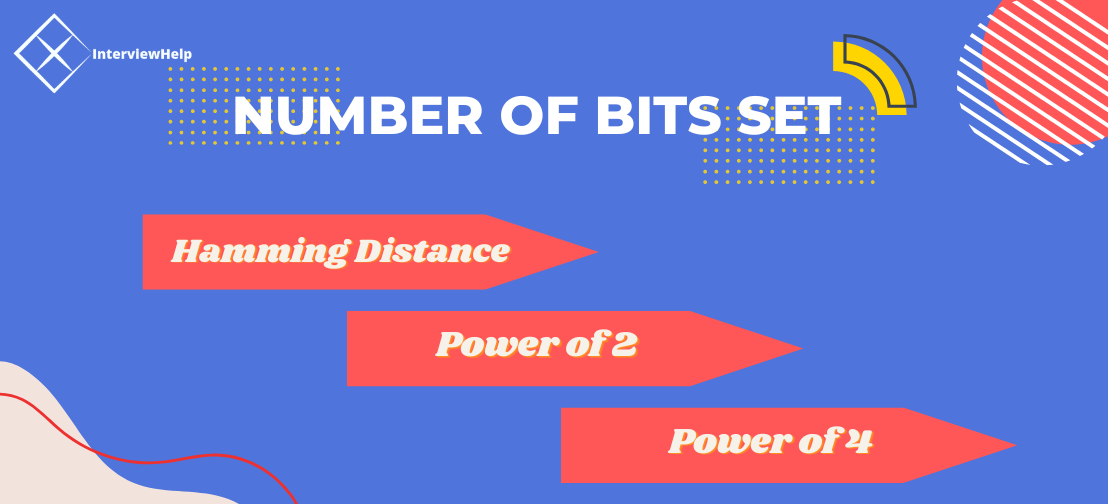# Number of Bits Set### Problem

Write a function that takes an unsigned integer and return the number of ‘1’ bits it has. -

``````Example 1:

Input: 5
Output: 2
Explanation: Integer 2 in binary is "00000000000000000000000000000101" which has a total of two '1' bits.

Example 2:

Input: 8
Output: 1
Explanation: Integer 4 in binary is "00000000000000000000000000001000" which has a total of one '1' bit.
``````

### Brian Kernighan’s Algorithm

Notice that for any integer `n`, doing a bit-wise AND of `n` and `n-1` has the effect of flipping the least-significant 1 bit in `n` to `0`. For example, `7 & 6 = 6` and `8 & 7 = 0`.

Our problem can be solved by turning off `n`'s set bits one bit at time:

``````def count_set_bits(x):
count = 0
while x:
x &= x - 1
count += 1
return count
``````

### Hamming Distance

The Hamming distance between two integers is the number of positions at which the corresponding bits are different. We can compute the hamming distance between two integers `x` and `y` by invoking `count_set_bits(x ^ y)`.

### Power of 2

Brian Kernighan’s Algorithm can also be used to check if a positive number is a power of 2. Notice that a positive integer is a power of `2` if and only if exactly one bit is equals `1` in its binary representation. So to check if positive integer `x` is a power of `2`, we can simply compare `x & (x-1)` with `0`:

``````def is_power_of_2(x):
"""Checks whether a positive integer is a power of 2."""
return x & (x-1) == 0
``````

### Power of 4

What about power of 4? An extension to the power of 2 question is checking whether a positive integer is a power 4.

For a positive integer `n` to be a power of `4`, it’s necessary that `n` be a power of `2`. What are some other requirements? Let’s write down some positive integers in their binary forms:

`````` 2:       10
4:      100
8:     1000
16:    10000
32:   100000
64:  1000000
``````

Notice that in order for `x` to be a power of `4`, its only set bit should be at the third, fifth or seventh, etc bit counting from the right. How do we check that? By creating a mask `0b01010101010101010101010101010101` which is `0x55555555` in hex:

``````def is_power_of_4(x):
"""Checks whether a positive integer is a power of 4."""